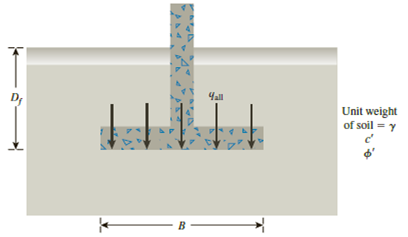Chapter 16, Problem 16.4PPrinciples of Geotechnical Enginee...

9th Edition
Braja M. Das + 1 other
ISBN: 9781305970939

Solutions

Chapter
SectionPrinciples of Geotechnical Enginee...

9th Edition
Braja M. Das + 1 other
ISBN: 9781305970939
Textbook Problem

Redo Problem 16.1 with the following: γ = 16.5 kN/m3, cu = 41 kN/m3, ϕ ′ = 0 , Df = 1.5 m, and factor of safety = 5.16.1 A continuous footing is shown in Figure 16.17. Using Terzaghi’s bearing capacity factors, determine the gross allowable load per unit area (qall) that the footing can carry. Assume general shear failure. Given: γ = 19 kN/m3, c′ = 31kN/m2, ϕ ′ = 28 ° , Df = 1.5 m, B = 2 m, and factor of safety = 3.5.Figure 16.17

To determine

Find the gross allowable load per unit area (qall) that the footing can carry.

Explanation

Given information:

The unit weight of the soil γ is 16.5kN/m3.

The value of uniformity coefficient cu is 41kN/m3.

The soil friction angle ϕ is 0°.

The depth of foundation Df is 1.5 m.

The factor of safety Fs is 5.

Calculation:

Determine the ultimate bearing capacity of the soil (qu) using the relation.

qu=cuNc+qNq=cuNc+γDfNq (1)

Here, Nc is the contribution of cohesion and Nq is the contribution of surcharge.

Refer Table 16.1, “Terzaghi’s bearing-capacity factors–Nc, Nq, and Nγ” in the textbook.

Take the Nc as 5.7 and Nq as 1.00 for the ϕ value of 0°.

Substitute 41kN/m3 for cu, 5

Still sussing out bartleby?

Check out a sample textbook solution.

See a sample solution

The Solution to Your Study Problems

Bartleby provides explanations to thousands of textbook problems written by our experts, many with advanced degrees!

Get Started

Solve Problem 20.1 using Excel.

Engineering Fundamentals: An Introduction to Engineering (MindTap Course List)

The Prius PHEV offers many advantages over the basic Prius, how is that possible?

Automotive Technology: A Systems Approach (MindTap Course List)

Discuss the importance of data models.

Database Systems: Design, Implementation, & Management

What e some of the benefits and shortcomings of using CASE tools for process modeling activities?

Systems Analysis and Design (Shelly Cashman Series) (MindTap Course List)

Name metal cutting machines used in the welding shop and their advantages.

Welding: Principles and Applications (MindTap Course List)

What three characteristics about TCP distinguish it from UDP?

Network+ Guide to Networks (MindTap Course List)